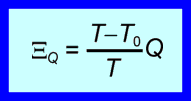# Sign Conventions for Heat and Work in Exergy Analysis

The ‘Positive in’ convention is best in exergy analysis

When the ‘Positive in’ sign convention is used, any exergy input to the system is positive and any work input is positive. The following expression describes the exergy transfer associated with shaft work at the boundary of a system.(1)

This expression indicates that the exergy transfer has the same direction as the shaft work.

When the ‘Positive in’ sign convention is used, any exergy input is positive and any heat transfer into the system is positive. The following expression describes the exergy transfer associated with heat transfer.(2)

This expression gives not only the magnitude of the exergy transfer, but also its direction. The direction of the exergy transfer depends on the temperature at which the heat transfer occurs relative to the temperature of the surroundings. If the heat transfer occurs at a temperature above the temperature of the surroundings, then the exergy transfer has the same direction as the heat transfer; otherwise it has the opposite direction.

## Inconsistencies of the ‘Positive heat in, positive work out’ convention

If the ‘Positive heat in, positive work out’ convention were to be applied, the first question to be answered would be ‘what sign convention should be used for exergy?’ Either way, there are significant inconsistencies. Should exergy transfer have a different sign convention to exergy transport? This too is a fraught question within the context of the ‘Positive heat in, positive work out’ convention.

The exergy transfer associated with shaft work at the boundary of a system has the same magnitude and direction as the shaft work itself. It may seem perfectly reasonable, therefore, to use the same sign convention for exergy transfer as for work: exergy transfer outwards would thus be positive according to the ‘Positive heat in, positive work out’ convention. Equation 1 above would be unchanged and there would be a negative sign in front of the right hand side of Equation 2. It should be noted that Equation 2 does not generally appear in the literature with a negative sign:the ‘Positive heat in, positive work out’ convention is tacitly ignored or overridden. To insert the negative sign would be counter-intuitive, as it would seem to suggest the wrong direction for the exergy transfer.

Is exergy, which is a co-property of a system and the surroundings, to be treated differently to other extensive quantities that can cross the boundary of a system, such as mass, internal energy and Gibbs free energy? In the steady flow energy equation, kinetic energy and potential energy that are transported across the boundary of a system are universally considered positive when they enter the system. It would seem logical to treat exergy transport across the boundary in the same way. However, doing this introduces an unnatural distinction between exergy transport (which is positive when into a system) and exergy transfer (which is positive when out of a system).

Suppose we stick with the ‘Positive heat in, positive work out’ convention, but define the inwards direction as the positive direction for exergy transfer or transport. We would then have a ‘Positive heat in, positive work out, positive exergy in’ convention. Equation 1 above would now have a negative sign before the W on the right hand side. As far as we known, this formulation has never been suggested. It would be counter-intuitive as it would seem to suggest that the exergy transfer was opposite to the work transfer.

The ‘Positive in’ convention works best, both for first law and second law analysis. It simplifies life. That’s why I prefer it.

Leo Nest, ULFC

<< Back to Sign Convention for Heat and Work